# Chain Length Problem in Radical Polymerization

In this note, I describe a statistical problem concerning the degree of polymerization of polymers formed through free radical polymerization, a possible model of the reaction mechanism, a simulation and why it fails.

Suggesting ideas on the improvement of the problem solving strategy or the model will be greatly appreciated

# Chain Length Problem

How does the distribution of the degree of polymerization (number of monomer units) of polyethene vary with the initial concentration of ethenes and the free radicals?

# Reaction Mechanism

## Chain Initiation

This is benzoyl peroxide.When heated, it breaks up into two benzoyl free radicals.The benzoyl further breaks up into a phenyl radical and carbon dioxide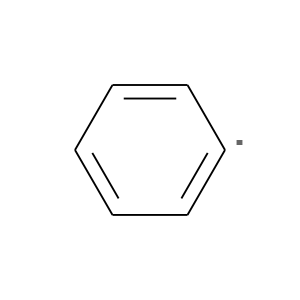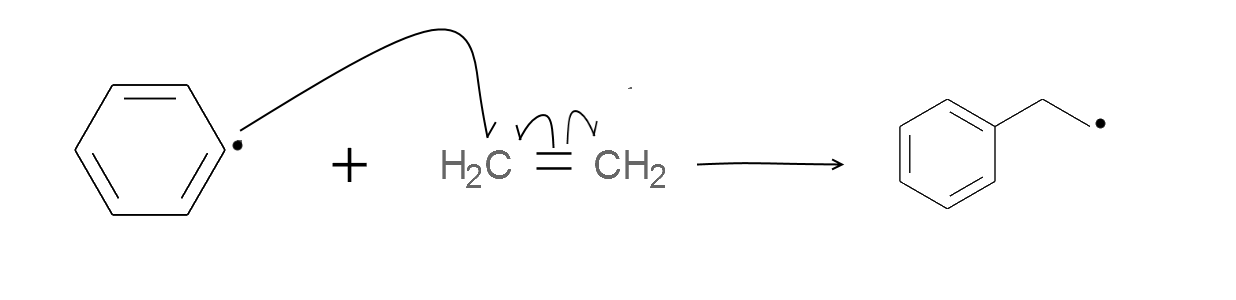## Chain Propagation

The free radicals keep attacking the ethenes and keeps growing.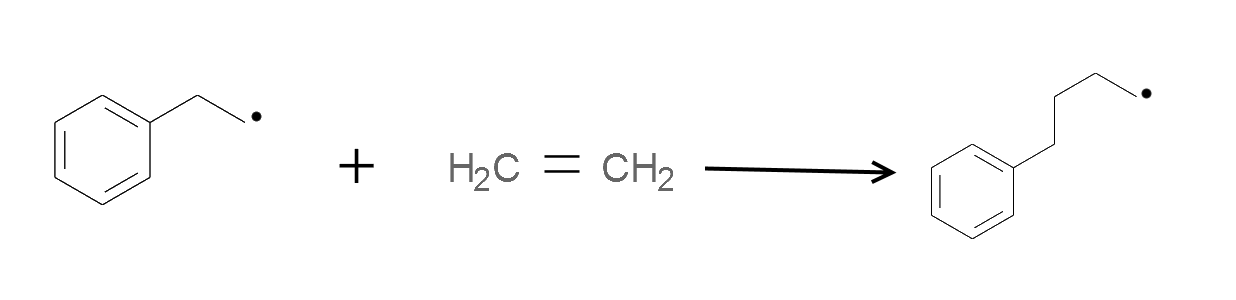## Chain Termination

The chain reaction terminates when the free radicals die out.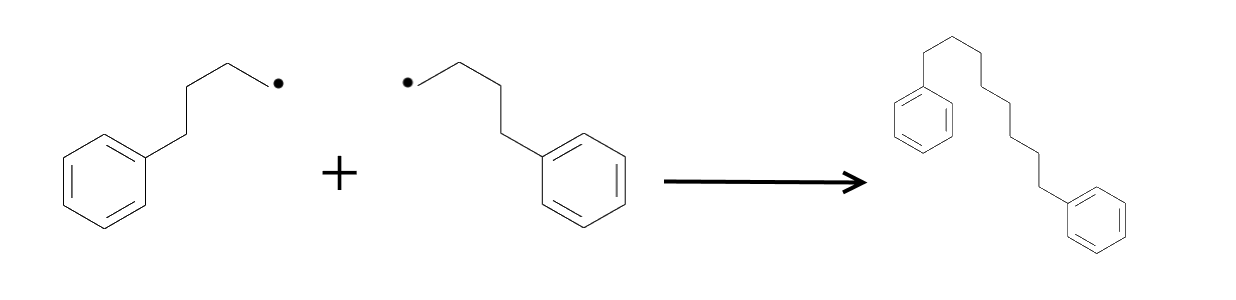For the purpose of the spherical cow model, we will assume that it is also possible that two phenyl radicals join to form biphenyl.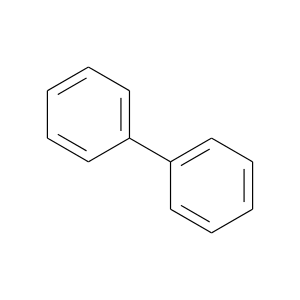It is evident that the reaction stops when there are no more free radicals.

# Agnishom's Spherical Cow Model of Polyethene Radical Polymerization

The spherical cow model is a simulation friendly model that tries to mimic the process of radical polymerization.

Parameters: The number of free radicals ($n_1)$) and the number of ethylene molecules ($n_2$) in the system.

The model only distinguishes between three kinds of species:

1. Free Radicals: Each free radical has a variable called chainLength, which is the number of monomer units attached to it.

2. Closed Chains: They also have the chainLength variable. However, they are incapable of further change.

3. Ethenes: They are just simple ethenes. All ethenes are identical and can be potentially attacked by a free radical.

The process of polymerization is modelled here as a process with discrete time steps during each of which two objects, chosen with uniform probability from the system, interact.

The interaction rules are as follows:

 Species Species Result Ethene Ethene Nothing happens Closed Chain (m) Closed Chain (n) Nothing happens Free Radical (m) Closed Chain (n) Nothing happens Ethene Closed Chain (n) Nothing happens Free Radical (m) Free Radical (n) Both radicals are removed. A new Closed Chain of length (m+n) is formed Free Radical (m) Ethene The ethene is removed. The size of the free radical increases by 1

# Monte-Carlo Simulation Approach

So far, the Monte Carlo Simulation (Code) was not very helpful because of the large number of steps involved in the actual reaction.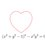5 years, 6 months ago

This discussion board is a place to discuss our Daily Challenges and the math and science related to those challenges. Explanations are more than just a solution — they should explain the steps and thinking strategies that you used to obtain the solution. Comments should further the discussion of math and science.

When posting on Brilliant:

• Use the emojis to react to an explanation, whether you're congratulating a job well done , or just really confused .
• Ask specific questions about the challenge or the steps in somebody's explanation. Well-posed questions can add a lot to the discussion, but posting "I don't understand!" doesn't help anyone.
• Try to contribute something new to the discussion, whether it is an extension, generalization or other idea related to the challenge.

MarkdownAppears as
*italics* or _italics_ italics
**bold** or __bold__ bold
- bulleted- list
• bulleted
• list
1. numbered2. list
1. numbered
2. list
Note: you must add a full line of space before and after lists for them to show up correctly
paragraph 1paragraph 2

paragraph 1

paragraph 2

[example link](https://brilliant.org)example link
> This is a quote
This is a quote
    # I indented these lines
# 4 spaces, and now they show
# up as a code block.

print "hello world"
# I indented these lines
# 4 spaces, and now they show
# up as a code block.

print "hello world"
MathAppears as
Remember to wrap math in $$ ... $$ or $ ... $ to ensure proper formatting.
2 \times 3 $2 \times 3$
2^{34} $2^{34}$
a_{i-1} $a_{i-1}$
\frac{2}{3} $\frac{2}{3}$
\sqrt{2} $\sqrt{2}$
\sum_{i=1}^3 $\sum_{i=1}^3$
\sin \theta $\sin \theta$
\boxed{123} $\boxed{123}$

Sort by:

Correct me if I'm wrong. Is the purpose of this code to calculate the number of types of phenyl - n chain - phenyl compounds that one can get from a given quantity of benzoyl peroxide?

If so, can one try a more "kinetic" approach using rate laws to solve the question?

- 5 years, 5 months ago

I think a continuous kinetic approach will work much better than my discretized approach. However, because my knowledge of chemistry is limited, I couldn't try that.

- 5 years, 5 months ago

I tried the case where biphenyls are the only product that can be formed. I think the rate of reaction for a 3 step biphenyl formation reaction is, r = k[benzoyl peroxide] (where the square brackets indicate concentration).

This was under the assumption that the unstable phenyl radical doesn't react with carbon dioxide.

I tried the case where (1,4- bi phenyl butane), (1,2-bi phenyl ethane), and biphenyl are the final compounds that are obtained.

I believe for the above case (1,4-biphenyl butane case) the rate law is,

r = K($\sqrt{(k[ethene])^{2} + 4q[benzoyl peroxide]} - k[ethene])^{2}$ where K,q,k are constants

This equation is for the rate at which biphenyl is formed in the second case. This was done just to see how the rate law will change as the chain terminates at different chain lengths.

When k[ethene] nears zero (the entire reaction resembles the 3 step reaction for the biphenyl), the rate law will accordingly change to match the first scenario's rate law.

- 5 years, 5 months ago

I think I am misconveying the question I want to ask. What I am interested in here is an idea of the length of the chains formed based on the initial concentrations of the materials.

- 5 years, 5 months ago

My idea was to use kinetics to determine the rate at which each chain is formed. So under certain conditions your program can eliminate the possibility of a certain product.

For example:

Lets say you use your program for the second case, when the concentration of ethene is very low (near zero). The rate at which biphenyl is produced is very large compared to the rates at which the other products are formed. Biphenyl will be the major product and its "chain_length" is 0.

In the same case, when the concentration of ethene is very very large compared to the concentration of benzoyl peroxide. The at which biphenyl is produced is almost negligible compared to rate at which the other two products. If one can determine their rate laws, your program can hopefully eliminate another compound (this might not be so. I don't know the rate laws, for all you know both of the other products might be favored). Hence the "chain_length" is 2 or 4 or both.

My idea was if we build more rate laws for cases where larger chains are involved and we see a pattern in them, we can assume the same situation for cases like phenyl - 100 carbon - phenyl. This generalized rate law for the entire mechanism can be applied in your program.

I worked out the rate laws for the case when (phenyl - 4 carbon - phenyl) , (phenyl - 2 carbon - phenyl), and biphenyl.

If you define $\alpha$ = (1/K)[$\sqrt{(k[ethene])^{2} + 4q[benzoyl peroxide]} - k[ethene]$]

then the rate at which biphenyl forms is:

$r_1$ = K$\alpha^{2}$

The rate at which phenyl - 2 carbon - phenyl forms is:

$r_2$ = p$\alpha$($\sqrt{r[benzoyl peroxide]}$ - l$\alpha$)

The rate at which phenyl - 4 carbon - phenyl forms is:

$r_3$ = m$(\sqrt{r[benzoyl peroxide]} - l(\alpha))^{2}$

where k,q,K,m,r,l are constants.

These equations suggest that in the second sub case the chain_length will be 4.

Now for this to really help (not just for specific cases) you need to integrate this expression. This is where we have to introduce the spherical cow. For the sake of simplicity, assume that the concentration of benzoyl peroxide is equal to the concentration of phenyl radicals. After integration you can vary the concentrations of the reactants and see how the the concentrations of products behave at a given instant.

- 5 years, 5 months ago

I see. The integration idea might be helpful here. Thank you!

- 5 years, 5 months ago

The integration seems like a difficult task, I tried it but I didnt get anywhere..

If you want the derivation for the rate laws, I'll email them. I didn't include them because I dont know how to add images in the comments.

- 5 years, 5 months ago

The syntax is ![description](URL)

- 5 years, 5 months ago

I created a note and have posted the pictures there. Here is the link:

I hope this helped.

- 5 years, 5 months ago

Could you modify the existing code to stop the reaction at (phenyl- 4 carbon - phenyl) and see how the results compare with the rate laws.

I found an interesting article related to this. A quicker approach

- 5 years, 5 months ago